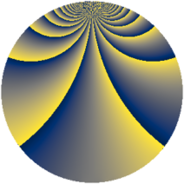# Properties

 Label 1470.2.bqLevel $1470$ Weight $2$ Character orbit 1470.bq Rep. character $\chi_{1470}(109,\cdot)$ Character field $\Q(\zeta_{42})$ Dimension $672$ Sturm bound $672$

# Related objects

## Defining parameters

 Level: $$N$$ $$=$$ $$1470 = 2 \cdot 3 \cdot 5 \cdot 7^{2}$$ Weight: $$k$$ $$=$$ $$2$$ Character orbit: $$[\chi]$$ $$=$$ 1470.bq (of order $$42$$ and degree $$12$$) Character conductor: $$\operatorname{cond}(\chi)$$ $$=$$ $$245$$ Character field: $$\Q(\zeta_{42})$$ Sturm bound: $$672$$

## Dimensions

The following table gives the dimensions of various subspaces of $$M_{2}(1470, [\chi])$$.

Total New Old
Modular forms 4128 672 3456
Cusp forms 3936 672 3264
Eisenstein series 192 0 192

## Trace form

 $$672q - 56q^{4} + 4q^{5} - 8q^{6} - 56q^{9} + O(q^{10})$$ $$672q - 56q^{4} + 4q^{5} - 8q^{6} - 56q^{9} + 2q^{10} - 60q^{11} + 4q^{14} + 10q^{15} + 56q^{16} + 36q^{19} - 20q^{20} - 8q^{21} - 4q^{24} + 2q^{25} + 32q^{26} + 16q^{29} - 12q^{31} + 32q^{34} + 20q^{35} - 112q^{36} + 104q^{39} + 26q^{40} - 40q^{41} + 60q^{44} + 52q^{45} + 4q^{46} + 92q^{49} + 16q^{50} - 104q^{51} - 4q^{54} + 106q^{55} - 48q^{56} + 100q^{59} + 26q^{60} - 104q^{61} + 112q^{64} + 24q^{65} + 16q^{66} + 48q^{69} + 40q^{70} - 16q^{71} - 32q^{74} + 8q^{75} - 40q^{76} - 12q^{79} + 4q^{80} + 56q^{81} - 16q^{84} - 64q^{85} - 192q^{86} - 8q^{89} + 4q^{90} + 20q^{91} - 48q^{94} + 212q^{95} + 4q^{96} - 8q^{99} + O(q^{100})$$

## Decomposition of $$S_{2}^{\mathrm{new}}(1470, [\chi])$$ into newform subspaces

The newforms in this space have not yet been added to the LMFDB.

## Decomposition of $$S_{2}^{\mathrm{old}}(1470, [\chi])$$ into lower level spaces

$$S_{2}^{\mathrm{old}}(1470, [\chi]) \cong$$ $$S_{2}^{\mathrm{new}}(245, [\chi])$$$$^{\oplus 4}$$$$\oplus$$$$S_{2}^{\mathrm{new}}(490, [\chi])$$$$^{\oplus 2}$$$$\oplus$$$$S_{2}^{\mathrm{new}}(735, [\chi])$$$$^{\oplus 2}$$